# Basic Arithmetic : Ratios and Rates

## Example Questions

← Previous 1 3

### Example Question #1 : Unit Multipliers

How many minutes are in a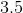hours?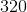minutes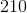minutes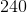minutes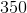minutesminutes

Explanation:

Knowing that there are 60 minutes in an hour, multiply that amount by the total number of hours (3.5):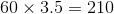minutes

### Example Question #1 : Ratios And Rates

If I wantyards of fabric, how many inches will I have?inches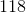inchesinchesinchesinches

Explanation:

1. Find out how many feet you have:

Knowing that there are 3 feet in a yard, you have 3 x 4 yards = 12 feet of fabric.

2. Find out how many inches you have:

Knowing that there are 12 inches in a foot, you have 12 x 12 inches=144 inches of fabric.

### Example Question #1 : Ratios And Rates

A football player ranyards with the football before he was tackled. How many feet did he run?Explanation:

Since there are 3 feet to a yard, multiply the number of yards by 3.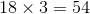### Example Question #1 : Ratios And Rates

How many days are in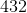hours?Explanation:

There are 24 hours in a day. To find out how many days are in 432 hours, divide that value by 24, the total number of hours in a day.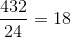### Example Question #1 : Unit Multipliers

How many centimeters are inmeters?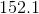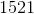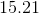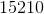Explanation:

There arecentimeters inmeter.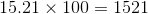### Example Question #2 : Unit Multipliers

How many inches are inyards?Explanation:

First, convert yards to feet.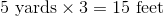Now, convert feet to inches.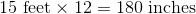### Example Question #3 : Unit Multipliers

How many hours are in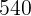minutes?Explanation:

Because there are 60 minutes in an hour, divide 540 by 60.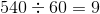### Example Question #4 : Unit Multipliers

Lucy measured that she needsfeet of wood to build her new bed. How many inches of wood should she buy?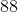Explanation:

To convert from feet to inches, multiply by 12.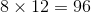### Example Question #2 : Unit Multipliers

How many minutes are there indays?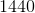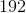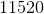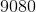Explanation:

First, convert the number of days into number of hours.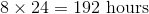Next, convert the hours into minutes.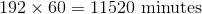### Example Question #2 : Unit Multipliers

How many feet are in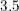miles?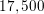feet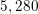feet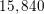feetfeet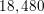feetfeet

Explanation:

When doing unit conversions, it's essential to know how many of the one unit equals how many of the other. In this case, we need to know that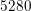feet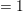mile. Therefore,miles equals: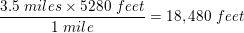Our final answer is thereforefeet.

← Previous 1 3

### All Basic Arithmetic Resources